# Calculating Napier's logarithm

We’ll show that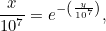The point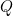moves at a constant speed of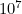, so we have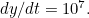Since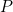moves at a speed that is proportional to the distance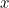left to travel we have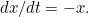From this we see thatwhich gives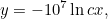for some constant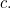We can work out the value ofusing our initial conditions. At the start, the pointstill needs to travel the whole length of the line segment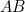, which is. Therefore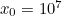. The pointhasn’t gone anywhere yet, so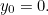Plugging this into the expression above gives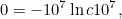so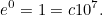Therefore,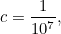so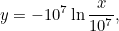andwhich is what we wanted to show.

Back to main article